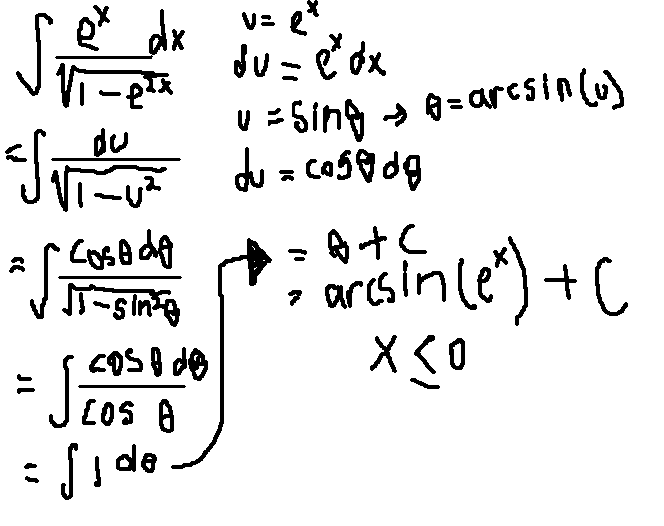# Famous arcsin formula

• Riazy
In summary, the conversation was about an integral with e^x divided by algebraic expressions and a variable switch. The solution involved using a famous arcsin formula and doing a substitution twice, with a restriction on x. The conversation also mentioned the use of u as e^x and sin x at the same time, and the importance of reading the entire integral before attempting to solve it.

$e^x / Sqrt[1-e^[2x]] (An integral with e^x div by algebraic expressions)(Scan paper) ## Homework Statement Ok I will try my best to write this with digital characters:$e^x / Sqrt[1-e^[2x]]
You will see the problem attached on an image below for more details.

e^x is present

## Homework Equations

When I do a variable switch, I recalled a famous arcsin formula (also listed below on my scanned paper)

## The Attempt at a Solution

See the picture below

[PLAIN]http://img839.imageshack.us/img839/2892/img0003001.jpg [Broken]

Last edited by a moderator:

You were right to think that it was an arcsin integral. This is how it is derived:

You have to do substitution it twice and there is a restricting on x.$$\int \frac{u}{\sqrt{1-u^2}} du$$

then just do another substitution like t=1-u2, which would make your integral much simpler.

rock.freak667 said:

$$\int \frac{u}{\sqrt{1-u^2}} du$$

then just do another substitution like t=1-u2, which would make your integral much simpler.

But if you do that, wouldn't there be a radical in the numerator?
If you let t = 1-u2
Then...

$$\int \frac{u}{\sqrt{1-u^2}} du$$ becomes $$\int \frac{\sqrt{t^2-1}}{t} du$$
Or am I missing something?

planauts said:
But if you do that, wouldn't there be a radical in the numerator?
If you let t = 1-u2
Then...

$$\int \frac{u}{\sqrt{1-u^2}} du$$ becomes $$\int \frac{\sqrt{t^2-1}}{t} du$$
Or am I missing something?

You'd need to get dt=2u du, such that u du=dt/2.

how did you make v = sin x = arcsin (v)

Because as far as I am concerned its not appearing in the integral yet

Wait.. Did you use U as e^x and both sin x at the same time?, both of them up there are u:s then?

Last edited:

Riazy said:
how did you make v = sin x = arcsin (v)

Because as far as I am concerned its not appearing in the integral yet

Wait.. Did you use U as e^x and both sin x at the same time?, both of them up there are u:s then?

Your integral contains an extra 'u' term in the numerator, so you can't really just use the result to get arcsine.

rock.freak667 said:
Your integral contains an extra 'u' term in the numerator, so you can't really just use the result to get arcsine.

And it should not contain that extra u term.

If you use u=e^x, then du=e^x dx

Your original integrand: e^x dx / (sqrt(1-e^2x))

So you get a new integrand: du / (sqrt (1-u^2)) ... which fits the formula from the back of your head. Planauts basically showed how that arcsin derivative was confirmed.

Next time I should read the entire integral to be done instead of the last one